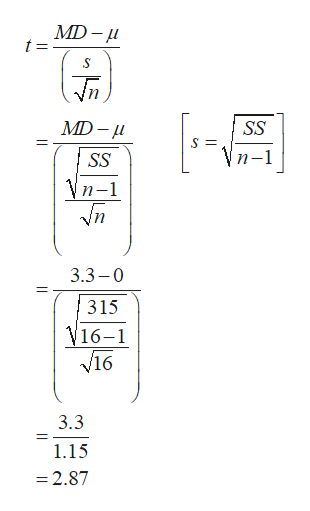# For a repeated measures study comparing two treatments with a sample of n=16 participants, a researcher obtains a sample mean difference of MD = 3.3 with SS = 315 for the difference scores. Calculate the repeated-measures t statistic for these data using a two-tailed test, and determine if it is enough to reject the null hypothesis. For this test use a=.01.

Question
8 views

For a repeated measures study comparing two treatments with a sample of n=16 participants, a researcher obtains a sample mean difference of MD = 3.3 with SS = 315 for the difference scores. Calculate the repeated-measures t statistic for these data using a two-tailed test, and determine if it is enough to reject the null hypothesis. For this test use a=.01.

check_circle

Step 1

The repeated measures t statistic is 2.87 and it is obtained below:

From the given information, the sample mean ...help_outlineImage TranscriptioncloseMD- t = S MD- SS п-1 SS Vn-1 3.3-0 315 V16-1 V16 3.3 1.15 =2.87 fullscreen

### Want to see the full answer?

See Solution

#### Want to see this answer and more?

Solutions are written by subject experts who are available 24/7. Questions are typically answered within 1 hour.*

See Solution
*Response times may vary by subject and question.
Tagged in

### Statistics